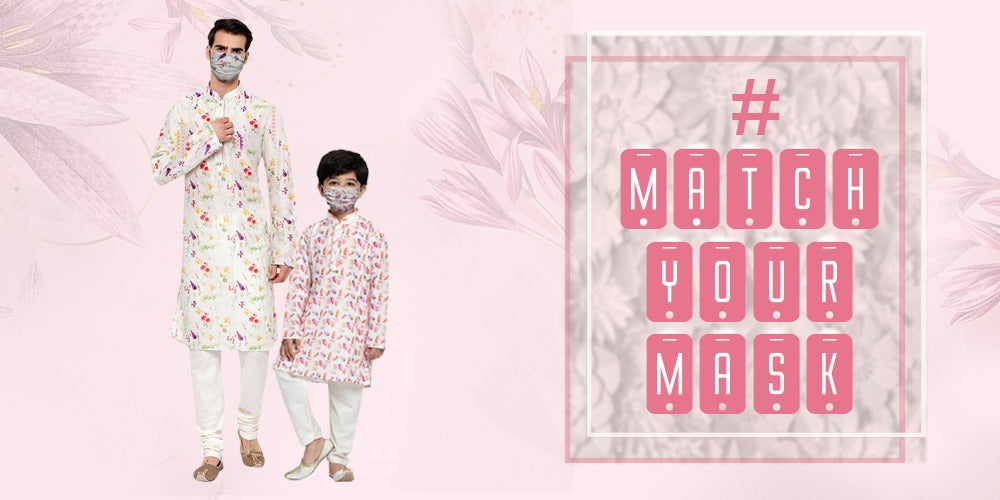Cash On Delivery Available

Free Shipping On Orders Above 1999/-

53 Results

Rs. 399.00

Unit price
per

Rs. 99.00

Unit price
per

Rs. 1,498.00

Unit price
per

Rs. 99.00

Unit price
per

Rs. 99.00

Unit price
per

Rs. 99.00

Unit price
per

Rs. 99.00

Unit price
per

Rs. 399.00

Unit price
per

Rs. 1,298.00

Unit price
per

Rs. 99.00

Unit price
per

Rs. 99.00

Unit price
per

Rs. 1,199.00

Unit price
per

Rs. 1,618.00

Unit price
per

Rs. 99.00

Unit price
per

Rs. 399.00

Unit price
per

Rs. 1,618.00

Unit price
per

Rs. 1,298.00

Unit price
per

Rs. 199.00

Unit price
per

Rs. 199.00

Unit price
per

Rs. 199.00

Unit price
per

Rs. 199.00

Unit price
per

Rs. 99.00

Unit price
per

Rs. 99.00

Unit price
per

Rs. 99.00

Unit price
per

Rs. 699.00

Unit price
per

Rs. 399.00

Unit price
per

Rs. 999.00

Unit price
per

Rs. 1,898.00

Unit price
per

Rs. 1,898.00

Unit price
per

Rs. 1,898.00

Unit price
per

Rs. 1,898.00

Unit price
per

Rs. 1,798.00

Unit price
per

Rs. 949.00

Unit price
per

Rs. 1,618.00

Unit price
per

Rs. 1,618.00

Unit price
per

Rs. 1,618.00

Unit price
per

Rs. 1,298.00

Unit price
per

Rs. 1,298.00

Unit price
per

Rs. 1,298.00

Unit price
per

Rs. 1,298.00

Unit price
per

Rs. 999.00

Unit price
per

Rs. 999.00

Unit price
per

Rs. 1,618.00

Unit price
per

Rs. 1,618.00

Unit price
per

Rs. 99.00

Unit price
per

Rs. 449.00

Unit price
per

Rs. 649.00

Unit price
per

Rs. 649.00

Unit price
per

Rs. 499.00

Unit price
per

#### VASTRAMAY Men's Pink Cotton Linen Blend Kurta

##### Vastramay

Rs. 649.00

Unit price
per